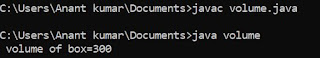## Wednesday, 4 July 2018

### User define function in C

A function can be define by the user as per the requirement of the user or programmer . To implement
a user define function , a programmer must contain the following three statement .

1. Function declaration or function prototype

2. Function call statement

3. Function definition

### # Function declaration or Function prototype :

The function provides the following information about a function .

A . What is return type of a function ? i.e which type of value is return by a function .

B . What is name of the function ?

C . How many argument or parameter is being used by a function ?

D . Type of argument or parameter being passed by a function ?

Syntax ;   return type function name ( data type 1 , data type 2 ) ;

Generally a function prototype is written before the definition of main function . It can also be written within main function . In this way it is written in two different ways and it is known as global or local. If it is written outside main function then it is called global function otherwise it is known as the local function prototype .

### # Function call statement :

A function call statement is an independent module which contains main statement which is written for the execution of program . This independent module is not part of the main function and always written out of the main function .

Syntax : A . Function call statement when a function return any value .

variable name = function name ( argument 1 , argument 2 ) ;

Syntax : B . Function call statement when a function do not return any value .

function name ( argument 1 , argument 2 ) ;

### # Function definition :

A function definition is that part of a program where the required exhaustible statement are written . It is main part of the program which is used to implement the concept of modularity in the program . This module is known as the user define function which is written or define as per the requirement of the user .

Syntax : return type function name ( data type argument 1 , data type argument 2 ) ;
{
statement ;
"
"
"
}

The function definition is always written out of the definition of main function .

EXAMPLE : Program calculate the addition of two number using function .

/* user define function */
# include <stdio.h>
int sum ( int , int ) ;                  /* function prototype */
void main ()
{
int a , b ;
clrscr () ;
printf ("\n Enter value of a:") ;
scanf ("%d", &a) ;
printf ("\n Enter value of b:") ;
scanf ("%d", &b) ;
c = sum ( a , b ) ;                              /* function call statement */
printf ("\n sum = %d", c) ;
getch () ;
}
int sum ( int a , int b )               /* function definition */
{
int c ;
c = a + b ;
return c ;
}

#### 1 comment:

1.Helpful for beginners progammer

## Featured post

### Program to find Volume of given box in java

class volume { public static void main (String args []) { int l=5,b=6,h=10; int volume; volume=l*b*h; System.out.println (" vol...